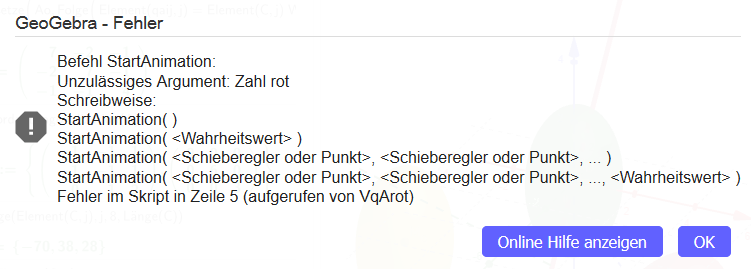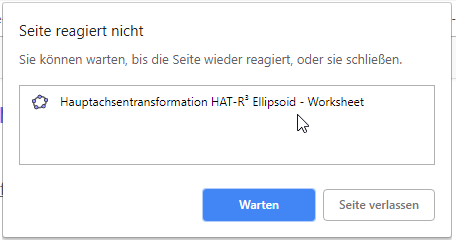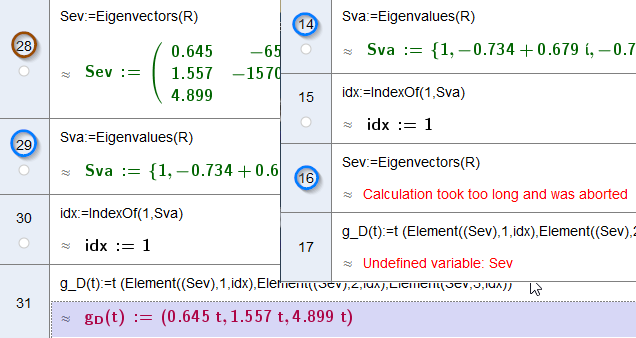hawe shared this problem 3 years ago
Solved

About history: Applet shows Error in Animation scriptSo I wrote a new script and give rot a new name. I had massive problems to upload the new applet and the Activity does not load orAny Ideas1

Sorry, https://www.geogebra.org/m/urk6rznt doesn't seem to have an object called "rot" now1

Yes, rot was a slider-object - rewirting the applet the slider name now is Rrot.

The error doesn't come up anymore, but the activitx sheet is dead - browser freeze the activity instance with the time out message box (chrome) ...1

I removed the animation part, but the applet did not load.

Time out1

The Applet seems to bee very unstable - testing various quadric-equations causes a complete break down - closeing app window - boom back to desktop.

The critical point seems to bee the command Eigenvectors. I hold 2 versions and in the work file the lines 28-29-30-31 has been placed to line 16,14,15,17 - a complet mess.

Eigenvector fails with too long calculation time (time out 1 min)The new placed lines disrupt the logical sequence of operations...

Any ideas?1

Does this work better? There were some problems in the XML with

`Evaluate(Evaluate(Evaluate(Evaluate(...`1

I've seen this evaluate chain in the construction protocol , it's quite normal when you use cas functions, isn't it..... There is a post from me in which I have pointed to this......

At the moment I only have my iPad to work with. The file opens and ggb has died, freeze until the app is closed after a while.

I have removed the eigenvector part and the applet has loaded, but I have a version with the eigenvector part running online in my workspace on the PC. What can I do to solve this problem?1

`I've seen this evaluate chain in the construction protocol , it's quite normal when you use cas functions, isn't it`

No, that shouldn't happen for newly created files in the current version. When did you start making this one?1

The quadric project I started these days, last week with the actual version ggb5 win10..

The first applet calculates a quadric transformation to normal form. then I started a new file with animation, got in trouble with gg6 online (rot error in animation script). because of the many different forms I started a 3. file to enable variable order of eigenvectors. user should be able to reproduce his specific calculation within the applet. i added a work around to extract the exact coefficients of the quadric because the command Coefficient kann handle conics only in algebraview. rounded values often produces a nonsense result.

i hope there's a way to make this project work, there's a lot of work involved.1

Something's wrong with this line (in HAT-EllipsoidR3_Bug.ggb)

`Q_M(Mq,aq):=Element({(x,y,z) Mq (x,y,z) } + { aq (x,y,z)},1);`

as these are different object types:

```(x,y,z) Mq (x,y,z)
aq (x,y,z)```

If you make your file without semicolons (;) I think it's easier to see where the problems are1

... and Coefficient(quadric) will work in the CAS from next release (v525)1

many thanks for your support. I have done some steps, but

q_A = (x,y,z) A (x,y,z) + a (x,y,z)=a_0

what is your proposal to calculate this equation? I have a terrible form to this (removed function).

I cut down the rotation matrix to 5 dezimals and this seems to help calculating the Eigenvectors: this part will calculate the rotation axis - that doesn't have to be so precisely..1

As a quick guess maybe you need to use this instead of (x,y,z)

`{x,y,z}`

or

`{{x},{y},{z}}`

but I don't understand what you are doing really11

My complet text in last comment has gone after editing - are you able to get it on reversion history or have I to write it again?11

OK, Math Background https://en.wikipedia.org/wi...

A = 3x3 matrix, a list of 3 elements, a_0 scalar value

(*)Quadric: q = (x,y,z) (A (x,y,z)) + a (x,y,z) = a_0

===> q:= (7 * x^(2)) + (7 * y^(2)) + (4 * z^(2)) - ((4 * x) * y) - ((2 * x) * z) - ((2 * y) * z) - (70 * x) + (38 * y) + (28 * z) = (-202)

(x,y,z) (A (x,y,z)) ===> (7 * x^(2)) + (7 * y^(2)) + (4 * z^(2)) - ((4 * x) * y) - ((2 * x) * z) - ((2 * y) * z)

a (x,y,z) ===> ((-70) * x) + (38 * y) + (28 * z)

Inline to gether

(x,y,z) (A (x,y,z)) + a (x,y,z) = a_0

===> something went wrong OR ?

For me it seems not to bee very clear what result I get out by vector/list product

See file Attachment lost Posting for more details...

I would appreciate it very much to learn how to calculate this term correctly and error-free and reliably!

To avoid conflicts list/vektor I have testet

Xq = {x², x y, x z, x y, y², y z, x z, y z, z²}

q=Simplify(Sum(Flatten(A) Xq )) + Sum(a {x,y,z}) = a_0

===>q: (7 * x^(2)) + (7 * y^(2)) + (4 * z^(2)) - ((4 * x) * y) - ((2 * x) * z) - ((2 * y) * z) - (70 * x) + (38 * y) + (28 * z) = (-202)

but this term changed object status Ellipsoid became Quadric and was lost any where - no instance in algebraview, no graphic output.

Nice suprise

Simplify(Sum(Flatten(D) Xq)) + Sum(a R {x,y,z}) -a_0=0

===>(9 * x^(2)) + (6 * y^(2)) + (3 * z^(2)) + ((54 * sqrt(2)) * x) + ((20 * sqrt(3)) * y) + ((4 * sqrt(6)) * z) + 1212 = 0

Something's going wrong, or what do you mean?1

So, I made a new version online

and I have tested many qadric forms. Summarizing I can say, that the applet run a reasonable job in windwos - ggb5 is more reliable as gg6 (eg. wrong rotation axis, classified zylinder as ellipsoid...see pic HATDiffRot).

The ipad App is not reliable. Calculation stop on different CAS lines, wrong classified quadrics - I made screeshots if it is of interest to you - Break down and freeze. Easy quadrics like Ellipsoid 1 / 4 x² + 4y² + (z - 3)² = 1 run quick and fine - but as soon as more complex equations come into play, there will be problems.

BTW: i was looking for the classic app for android but didn't find it - is it no longer available?

It is possible to switch clipping on/off - I have not found a script command?1

v525 is online now with Coefficients(conic / quadric) working in CAS (and some other small fixes)1

Many thanks for the hint...

I am try,

but matrix vector list product (quadric: (x,y,z) (A (x,y,z)) + a (x,y,z) = a_0) did not work (addition in a line) it is hard to belive that it is a problem to compute this quadric equation?1

Thanks, this will work in the next release (v527). In the meantime you'll need to split it over two cells:

`(x,y,z) (A (x,y,z)) + a (x,y,z)`1

Great that this will be fixed. Many, many Thanks...

An other example computing eigenvector

A:={{1, 1, 0}, {1, (-1), (-2)}, {0, 1, 1}}

Eigenwerte:={0, 1}

0 is double ===> to find an eigenvector basis I have to solve

Aλ1:=Solutions((A - Eigenwerte(1) Identity(n)) {x, y, z},{x, y, z})

EV1:={{1, (-1), 1}}

and

(A - Eigenwerte(1) Identity(n)) {x,y,z}

>{x + y, x - y - (2 * z), y + z}

Flatten(EV1)

>{1,-1,1}

but

(A - Eigenwerte(1) Identity(n)) {x, y, z} - Flatten(EV1)

>?